# PSEB 5th Class Maths Solutions Chapter 11 Patterns Ex 11.1

Punjab State Board PSEB 5th Class Maths Book Solutions Chapter 11 Patterns Ex 11.1 Textbook Exercise Questions and Answers.

## PSEB Solutions for Class 5 Maths Chapter 11 Patterns Ex 11.1

1. Study the patterns carefully and fill the boxes :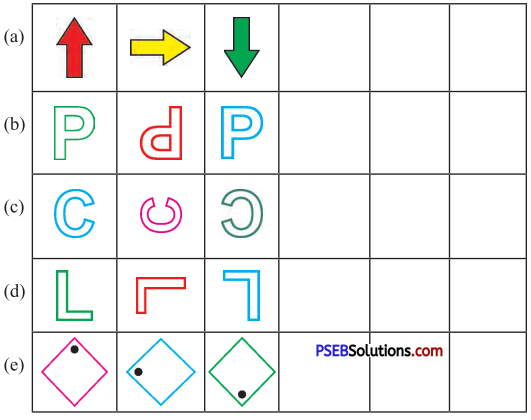Solution: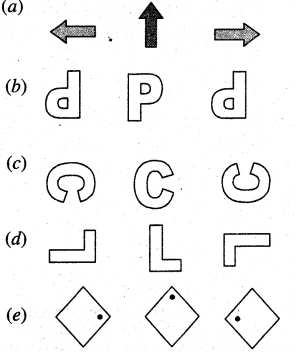2. See the given pattern and write the next two terms :

(a) (9 – 1) ÷ 8 = 1
(98 – 2) ÷ 8 = 12
(987 – 3) ÷ 8 = 123
Solution:
(9876 – 4) ÷ 8 = 1234
(98765 – 5) ÷ 8 = 12345(b) 9 × 9 + 7 = 88
98 × 9 + 6 = 888
987 × 9 + 5 = 8888
Sol.
9876 × 9 + 4 = 88888
98765 × 9 + 3 = 888888

3. Make triangular patterns with the help of the following numbers :

(a) 15
Solution: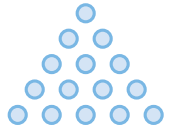1 + 2 + 3 + 4 + 5 = 15

(b) 21
Solution: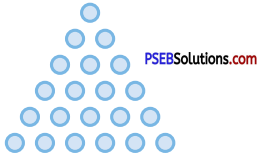1 + 2 + 3 + 4 + 5 + 6 = 21

Question 4.
Make dotted patterns using square numbers 5 × 5 = 25 and 6 × 6 = 36.
Solution: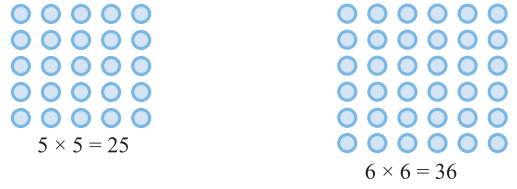Question 5.
Fill the numbers from 21 to 29 in such a manner In the given box that sum of rows/columns is 75.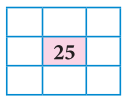Solution: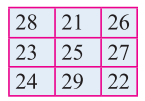Question 6.
Fill the following hexagon in such a way that number which comes in square must be the multiplication of its adjoining two circles.
a)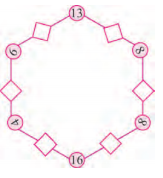Solution: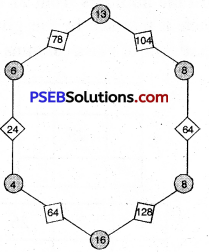b)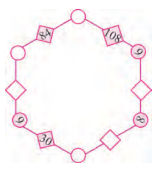Solution: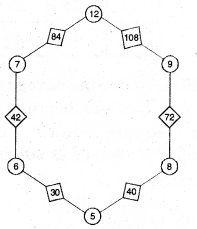Question 7.
Look at these number series and complete the blanks.
(a) 6, 13, 20, ………., …………, ………….
(b) 86, 80, 74, ………., …………, ………….
(e) 1600, 800, 400, ………., …………, ………….
(d) 28Z, 27Y, 26X, ………., …………, ………….
(e) ED, FE, GF, ………., …………, ………….
(f) 8, 108, 208, ………., …………, ………….
(g) 2, 6, 18, ………., …………, ………….
(h) 1, 8, 27, ………., …………, ………….
(j) 729, 243, 81, ………., …………, ………….
Solution:
(a) 27, 34, 41
(b) 68, 62, 56
(e) 200, 100, 50
(d) 25W, 24V, 23U
(e) HG, IH, JI
(1) 308, 408, 508
(g) 54, 162, 486
(h) 64, 125, 216
(i) 27, 9, 3.